# Number Sequences and Patterns

### Number Sequences are lists of numbers that form a relationship with each other and are connected in some way. They follow a pattern which you have to identify to extend the list of numbers or fill in the blanks.

Each number in the sequence is called a 'term' - so in the sequence 5, 6, 7, 8 ...
5 is the 1st term, 6 is the 2nd term, 7 is the 3rd term and so on.

Every sequence has a 'rule', once you discover the rule, you can use it to work out the missing numbers. For the sequence 1,2,3,4,5 ... the rule would be 'plus 1', so the next number would be 5 + 1 = 6, next is 6 +1 = 7 and so on.

There are several types of sequences and we will look in depth at the common types.

## Odd and Even

(check that your child can identify odd and even numbers
odd end in 1,3,5,7,9 - even end in 2,4,6,8,0)

### 5, 7, 9, 11, 13, _ , _ ... This is a sequence of odd numbers.  What are the next two numbers in the sequence ..... 15 and 17

Sequences can also go in reverse 22, 20, 18, 16, 14, _ , _ - rule is 'even numbers decreasing'

## Arithmetic Sequence

An Arithmetic Sequence either adds or subtracts a value to the previous term.

1, 5, 9, 13, 17, 21, 25, _ , _ ... Rule is 'add 4'

A useful strategy for dealing with sequences is to write them down, giving enough space to draw and label the hops from one term to another.

#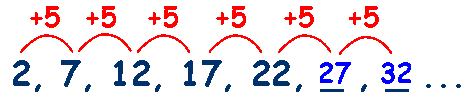Sometimes you will be asked to find a term at the beginning or in the middle of the sequence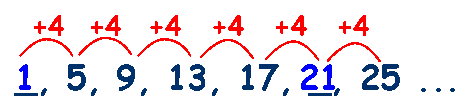and you will have to work backwards to find the missing term

## Geometric Sequence

A Geometric Sequence is when the previous term is multiplied or divided by a value.

2,4,8,16,32,64, _ , _ ... Rule is 'multiply by 2' or double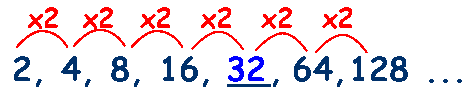## Changing Number

This is where the value changes with each step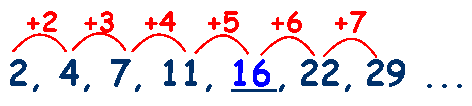## Repeated Pattern

This is where the pattern is repeated after a certain number of steps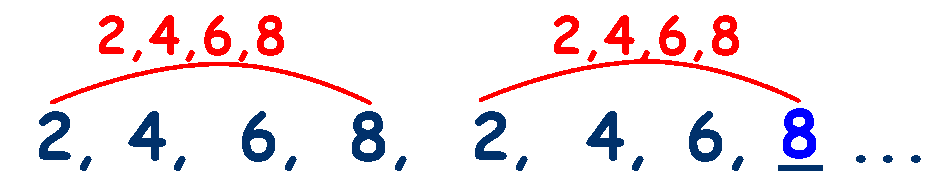## Triangular Numbers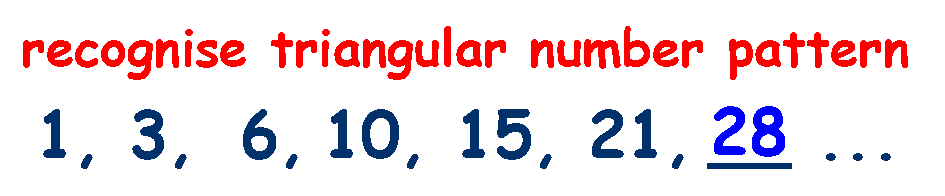## Square Numbers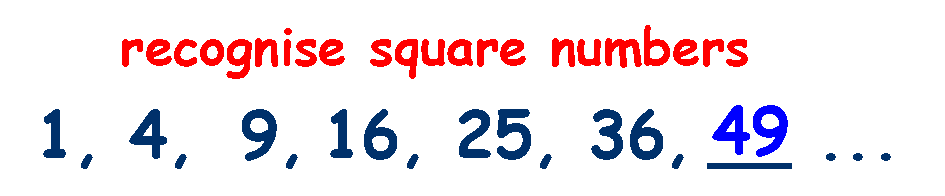## Cube Numbers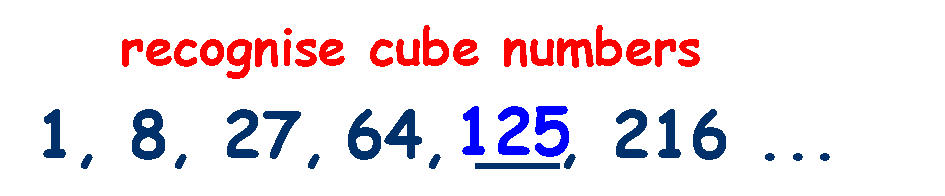## Fibonacci Numbers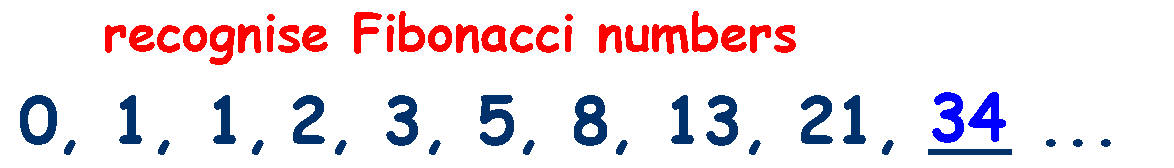Rule is: The number is found by adding the two previous terms.
(Term 5 is found by adding term 3 to term 4 ... 1 + 2 = 3)

This idea of sequences is worth spending the time on and making sure that your child has a good understanding of how to solve this type of question because it is used extensively in the Verbal Reasoning section of the 11 plus.

## Use number patterns and sequences to find  the Mission 2110 codes

### Play in full screen